# SAT II Math I : Solving Inequalities

## Example Questions

### Example Question #1 : Solving Inequalities

Give the solution set of the inequality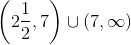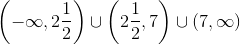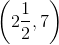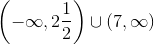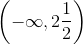Explanation:

Two numbers of like sign have a positive quotient.

Therefore,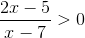has as its solution set the set of points at which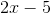and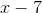are both positive or both negative.

To find this set of points, we identify the zeroes of both expressions.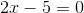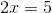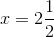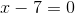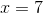Since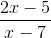is nonzero we have to excludeis excluded anyway since it would bring about a denominator of zero. We choose one test point on each of the three intervals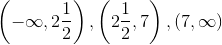and determine where the inequality is correct.Choose: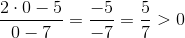- True.Choose: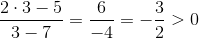- False.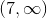Choose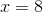: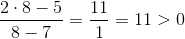- True.

The solution set is### Example Question #2 : Solving Inequalities

Solve for x.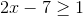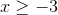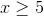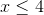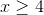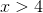Explanation:

Solving inequalities is very similar to solving an equation. We must start by isolating x by moving the terms farthest from it to the other side of the inequality. In this case, add 7 to each side.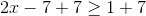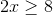Now, divide both sides by 2.### Example Question #3 : Solving Inequalities

Solve for x.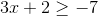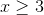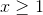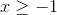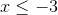Explanation:

Solving inequalities is very similar to solving an equation. We must start by isolating x by moving the terms farthest from it to the other side of the inequality. In this case, subtract 2from  each side.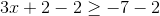Now, divide both sides by 2.### Example Question #2 : Solving Inequalities

Solve the following inequality: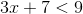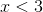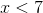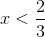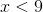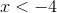Explanation:

To solve for an inequality, you solve like you would for a single variable expression and getby itself.

First, subtractfrom both sides to get,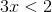.

Then divide both sides byand your final answer will be,.

### Example Question #5 : Solving Inequalities

Solve the inequality: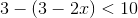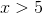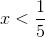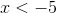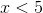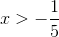Explanation:

Simplify the left side.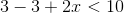The inequality becomes: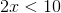Divide by two on both sides.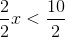The answer is:### Example Question #6 : Solving Inequalities

Solve the inequality: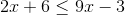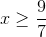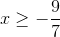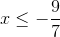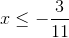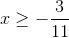Explanation:

Subtracton both sides.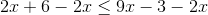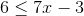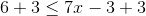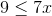Divide by 7 on both sides.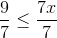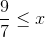The answer is: